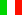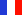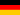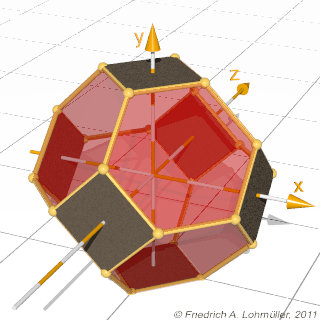###### Descriptions and Examples for the POV-Ray Raytracerby Friedrich A. Lohmüller Elementary Geometry for Raytracing
ItalianoFrançaisDeutschHome
- POV-Ray Tutorial

- Geometrical Basics
for Raytracing

Right-angled Triangle
Pythagorean Theorem
Trigonometry Basics
Law of cosines
Equilateral Triangle
Regular Polygon
Polyhedron
Tetrahedron
Octahedron
Cube & Cuboid
Dodecahedron
Icosahedron
Cuboctahedron
Truncated Octahedron
Rhombicuboctahedron
Truncated Icosahedron
Circles
Tangent circles
Internal Tangents
External Tangents

- Geometric 3D Animations## Truncated OctahedronSome useful geometrical facts.In the following we write for the square root of a number the expression "sqrt(ZAHL)"
conforming to the syntax used in POV-Ray.

 Dimensions Length of an edge: a. The radius of circumsphere: R = a / 2 * sqrt( 10 ); The radius of edgesphere (tangent to edges): Re = a * 3/2; The angle between hexagon and hexagon: ~ 109,471° Face_Angle = degrees(acos(-1/3)); The angle between hexagon and square: ~ 125,26° Edge_Angle = degrees(acos(-1/sqrt(3)));Truncated OctahedronTruncated Octahedron Tesselation 3DZeolith AZeolith A
top

 © Friedrich A. Lohmüller, 2011 http://www.f-lohmueller.de